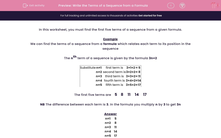# Write the Terms of a Sequence from a Formula

In this worksheet, students find the first five terms of a sequence from a given formula.Key stage:  KS 2

Curriculum topic:   Maths and Numerical Reasoning

Curriculum subtopic:   Sequences

Difficulty level:#### Worksheet Overview

In this worksheet, you must find the first five terms of a sequence from a given formula.

Example

We can find the terms of a sequence from a formula which relates each term to its position in the sequence

The nth term of a sequence is given by the formula 3n+2

 Substitute n=1 first term is 3×1+2 = 5 n=2 second term is 3×2+2 = 8 n=3 third term is 3×3+2 = 11 n=4 fourth term is 3×4+2 = 14 n=5 fifth term is 3×5+2 = 17

The first five terms are    5    8    11    14    17

NB The difference between each term is 3. In the formula you multiply n by 3 to get 3n